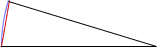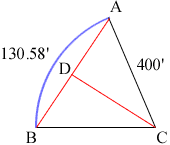Quandaries and Queries Can you please help me solve this problem: When Radius=400.00' and Arc=130.58' what is the Cord distance in feet? Thank you very much. David Hi David, I first drew a diagram, very roughly to scale, and I can see immediately that the answr is just a little less than 130.58"To describe a solution I need to distort the scale.In the diagram D is the midpoint of the chord AB so angle ADC is a right angle. The length of an arc is given by a = r theta where r is the radius of the circle and theta is the angle measured in radians. Thus, for your problem angle BCA = 130.58/400 = 0.32645 radians hence angle DCA = 0.32654/2 radians. But triangle DCA is a right triangle so sin( 0.32654/2) = |AD|/400. Solve for |AD|. Penny Go to Math Central